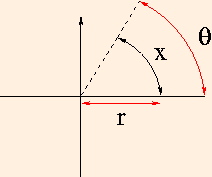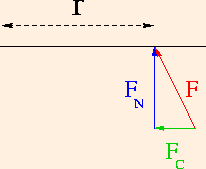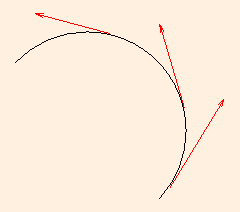# Rotational Motion

The analysis of linear motion which we have just completed has application in the movement of every organism in the animal kingdom. By contrast, there seems to be little use for rotation: very few animals revolve. But every animal with articulated joints participates in some measure in rotational motion: the movement of one bone relative to another to which it is joined is a partial rotation about the axis located at the joint. Whenever you walk, swing your arms, throw a ball or turn your head, you are moving rotationally.

### Uniform Circular Motion

Consider a pitcher throwing a ball in a game of fast pitch softball. We choose this example because the motion of the ball around the joint at the shoulder is very nearly circular, at least until the ball is released. As you can imagine, circular motion is the most simple kind of rotational motion: it is the rotational analog of one-dimensional linear motion. In keeping with our use of the point particle approximation, we will model the windup before the pitch as the circular motion at constant speed of a point object (the ball) about a fixed axis (the shoulder joint) in a plane.

Let us first examine the kinematics of the motion. While the ball is assumed to be moving at constant speed, it is most certainly not moving with constant velocity since the direction of the ball's motion is constantly changing as it moves around the circle. In this example, there are only two degrees of freedom: the radius of the circle and the speed of the ball. The centripetal acceleration experienced by the ball is a function of these two variables, and keeps the ball in a circular path.

When the ball is released it moves along a straight line tangent to the circle at the point of release. In fact, at any point along the circular motion, the direction of the velocity is tangent to the circle. This means that the direction of the centripetal acceleration is inward, toward the center of the circle. The centripetal force causing this acceleration must then be an attractive tension connecting the ball and the shoulder joint: it constrains the ball to move in a circle. Because the arm is a radius of the circle, we say that both the acceleration and the force are radial.

### General Rotational Motion

Let us now consider a small arc of the circle, and relax the condition that the ball be moving at a constant speed. Since the radius of the arc is a constant, there is a direct geometric relationship between the length of the arc and the angle subtended by it. Denoting this angle, or more properly, angular position, by θ, we have
x = r θ.Because the radius is constant, we also have a simple relationship between the velocity of the ball and the rate of change of the angle, called the angular velocity and denoted by the Greek letter omega:
v = r ω.
Similarly, the relationship between the acceleration of the ball and the angular acceleration is
a = r α,
where α is the Greek letter alpha. Since angle is the ratio of arc length to radius, angles carry no units. Hence the S.I. units of ω are 1/s and those of α are 1/s2. As in the linear case, we will assume that angular acceleration is always constant.
θ = θ0 + ω0 t + αt2 / 2.
Since this equation looks exactly like the position equation with a simple substitution of variables, we say that these two equations are isomorphic: they have the same shape. In fact, the equations of rotational motion are all isomorphic to their linear counterparts. This isomorphism is possible because linear motion and rotational motion around a circle are both one-dimensional: angular position is just as good a variable as linear position.

Perform the following experiment right now, while you are reading this text. Stand holding your loaded book bag by its straps loosely at your side. Keeping your arm stiff, slowly raise your book bag to shoulder level, if you can. Now loop the straps around your arm at the elbow joint and slowly raise the book bag to the same height. It is almost painfully obvious that less effort was required to lift the bag when it was closer to your shoulder. It is equally obvious that less effort is required when the bag is less full. Generalizing, we see that the rotational inertia of an object depends not only on its mass but also on the radius of its rotational motion. In this context, the radius is often called the lever arm.

Consider the force involved in this experiment. Since this experiment took place in two dimensions (as circular motion), the force may have any direction in the x-y plane. Taking the lever arm to extend along the x axis from the origin, we see that an arbitrary force may have independent x and y components. These are identified by drawing a right triangle whose hypotenuse is the force, and whose other sides are parallel to the x and y axes. The y component is labeled here as FN: the component normal to the lever arm. The x component is labeled as FC: the component parallel to the lever arm might act to compress it, but will not affect the rotation:τ = r FN.
τ = I α,
where I denotes the rotational (moment of) inertia. But we have just seen that torque has S.I. units of Newton meters, or kg m2/s2.
Note that while at the fundamental level these are units of energy, torque is not energy.
This implies that I has units of kg m2; since we know that rotational inertia depends on both mass and radius, we must have
I = m r2
for a point particle rotating around a circle. For more complex objects we need calculus in order to split the objects into infinitesimal pieces and add together the rotational inertia of all of the pieces.

You need a Java-capable browser to be able to use the applets. If they do not work with your Windows system, download the Java VM (Virtual Machine) for your version of Windows at the download section at java.sun.com.

You need a Java-capable browser to be able to use the applets. If they do not work with your Windows system, download the Java VM (Virtual Machine) for your version of Windows at the download section at java.sun.com.

Consider a car following a sharp turn to the left at speed. During the turn, the car is in circular motion: it follows an arc on a circle. At every point along that arc, its velocity vector is tangent to the arc. So, too, are the velocity vectors of everything in the car: an object dropped from the car during the turn would move in a straight line along the tangent at that point on the arc:The moving car constrains you to follow the arc, and the "force" you feel which seems to push you to the right is nothing more than your tendency to follow the tangent. From the point of view of an external observer, the only force you experience points toward the center of the arc: it is the centripetal force, here exerted by the friction between the tires and the road surface, which keeps the car moving along the arc.

In the next section we will use the tools of rotational motion to analyze your body in equilibrium: when forces are present but nothing moves.

©2008, Kenneth R. Koehler. All Rights Reserved. This document may be freely reproduced provided that this copyright notice is included.

Please send comments or suggestions to the author.